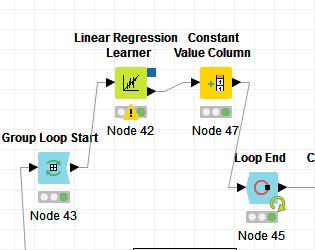# How to get R Square value of a 'Linear Regression Learner' node running in loop

I am running a Linear Regression through ‘Linear Regression Learner’ node in a ‘Group Loop’.
I am getting the intercept and coefficients but not the R squared value. How can I get the same for every iteration. Below is the image of the loop. I am using ‘Constant Value’ node to get the ‘groupIdentifier’ Flow variable.Hi @niki,

To get the R^2 measure you will need to score your model, which has to be done by evaluating it on test data, having preliminary split your data on a train and a test sets using the Partitioning node:

GettingR.knar.knwf (11.6 KB)

1 Like

Hi @amartin

I had implemented something of this sort earlier but it was very clumsy so checked if there is any better way. I don’t have much data so instead of using test data for prediction i used the train data itself for R^2 value.
Another issue I am facing is the R^2 values I got from a R tool based lm() function is very different from what I got in KNIME. I tried to redevelop my R tool model in KNIME to test KNIME’s capabilities. If the R^2 values of R tool and KNIME are different by definition?Hi @niki,

R^2 values as well as coefficients and intercept for the Linear Regression model in KNIME and with R should be the same.

Can you check how you calculate R^2 in R please?

I just check and for me they are the same:

Best,
Anna

@amartin

Can you please check the screenshots I shared for both KNIME workflow and output and suggest where could be the possible issue. Do I need to share more info?
I am getting intermittent rows where the R^2 of R tool and KNIME is same but that is just 6% of the total instances. Even for those the coefficients are not exactly same.

Hi @niki,

I am afraid it is not possible to check your workflow from the provided screenshot. You can attach the workflow and I’ll try to help. Also, I have to know which exactly R functions you’re using to train your model and to calculate the R^2.

What R tool are you using? You can also use the R Snippet node in KNIME.

Best,
Anna

How can I get the .knwf file?

Export your workflowhttps://www.knime.com/knime-introductory-course/chapter1/import-export-workflows

Br,
Ivan

Hi @ipazin

I used R studio to develop the model. Attaching the screenshot of code lines for reference.

I know a little bit of R but I wouldn’t get involved. Only helped with workflow export. Sry